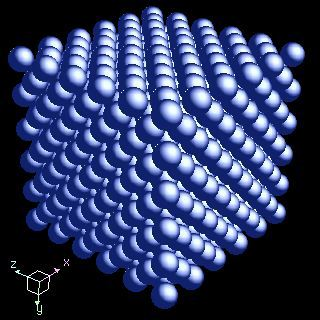Question #2b520

Mar 27, 2016

The coordination number of an atom in a crystal is the number of its "nearest neighbours".

Explanation:

The atoms in crystals are arranged in various repeating three-dimensional arrays.The atoms have different numbers of nearest neighbours, depending on how they are packed in the crystal.

The coordination number (CN) is a measure of how tightly the atoms are packed together.

Here are some examples.

The atoms in polonium ($\text{Po}$) are arranged at the corners of a cube.(from 2012books.lardbucket.org)

An atom in a polonium crystal has CN = 6.

You can see its six nearest neighbours in the diagram above.

The crystal structure of sodium ($\text{Na}$) has an additional atom in the centre of the cube (a body centred cube)An atom of $\text{Na}$ has CN = 8.

Its nearest neighbours are the 8 atoms at the corners of the cube.

The atoms in magnesium ($\text{Mg}$) are packed on top of each other like the apples in a crate (in a hexagonal closest packed structure).(from www.slideshare.net)

Each atom of magnesium has 12 nearest neighbours, so CN = 12.

The University of Sydney has some great animations that might help you visualise some crystal structures and their coordination numbers.

The video below shows how to find coordination numbers.